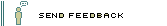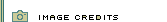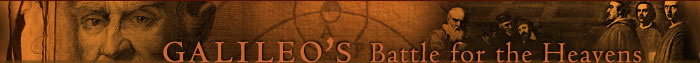His Experiments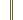Galileo homepage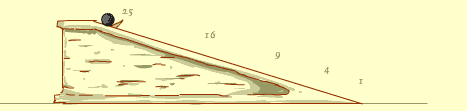That's right.

The distance that the ball travels, when starting from rest, is equal to the square of the time—it took 2 units of time to travel 4 units of distance, 3 units of time to travel 9 units of distance, etc. This could be written as d = t2, where d represents distance and t represents time. In this experiment, however, we chose distances that would give us round numbers when plugged into the formula. In real life, a constant needs to be added to the formula to make it work with measurements that we use (e.g., feet and seconds). This constant is A, which represents the rate of acceleration due to earth's gravity. The complete formula is d=1/2At2.

This wraps up the Inclined Plane experiment, but there are other Galileo experiments to explore. Make another selection.